Sie sind hier: ICP » R. Hilfer » Publikationen

# 5 Approximate Numerical Solution

[page 2333, §1]

## 5.1 Initial conditions

[2333.1.1] The preceding theoretical considerations are confirmed by numerical solutions of eq.  (23) with a simple jump-type hysteresis, as formulated in eq.  (29). [2333.1.2] The initial conditions are monotone and of the form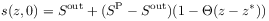(41)

where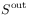is given in Table 1. [2333.1.3] Note that all initial conditions are monotone and do not have an overshoot. [2333.1.4] Two cases are, labelled A and B, will be illustrated in the figures, namely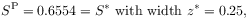(A)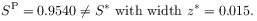(B)

[2333.1.5] Case A is chosen to illustrate travelling wave solutions with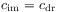. [2333.1.6] The second initial condition illustrates a general overshoot solution with two wave speeds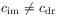. [2333.1.7] Moreover it illustrates one possibility for the limit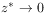and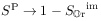in which a very thin saturated layer (that may arise from sprinkling water on top of the column) initiates an overshoot profile. [2333.1.8] This could provide a partial answer to the question of how saturation overshoot profiles can be initiated.

## 5.2 Numerical Methods

[2333.2.1] The numerical solution of eq.  (29) was performed using the open source toolkit for computational fluid mechanics Open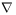FOAM . [2333.2.2] This requires to develop an appropriate solver routine. The solver routine employed here was derived from the solver [page 2334, §0]    scalarTransportFoam of the toolkit. [2334.0.1] Two different discretizations of eq. (29) have been implemented. One is based on a direct discretization of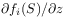using the fvc::div-operators, the other on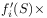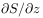using fvc::grad-operators. [2334.0.2] For the discretization of the time derivatives an implicit Euler scheme was chosen, for the discretization ofan explicit least square scheme, and for the discretization of the second order term an implicit Gauss linear corrected scheme has been selected. [2334.0.3] The second order term had to be regularized by replacing the function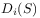with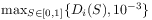. [2334.0.4] This avoids oscillations at the imbibition front. [2334.0.5] The discretized system was solved with an incomplete Cholesky conjugate gradient solver in OpenFOAM . [2334.0.6] The numerical solutions were found to differ only in the numerical diffusion of the algorithms. [2334.0.7] The divergence formulation seems to be numerically more stable and accurate.

## 5.3 Results

[2334.1.1] Equation (38) can have one solution, several solutions or no solution. [2334.1.2] This can be seen numerically, but also analytically. [2334.1.3] Depending on the values of the parameters the velocity of the imbibition front may be larger or smaller than that of the drainage front. [2334.1.4] With the parameters from Table 1 the overshoot saturation for a travelling wave as computed from (38) is found to be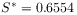and its velocity is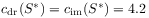.

[2334.2.1] The travelling wave solution expected from the graphical construction in Figure 1a) and b) for the initial condition A with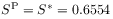and width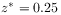is displayed in Figure 1c) at the initial time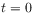and after dimensionless time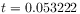corresponding to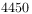s. [2334.2.2] It confirms a travelling wave of the form (36) whose velocity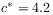agrees perfectly with that predicted from eq.  (38). [2334.2.3] We have also checked that the solution does not change with grid refinement.

[2334.3.1] For different initial conditions the numerical solutions also agree with the theoretical considerations. [2334.3.2] Saturation overshoot is found also when initially a thin saturated layer with steplike or linear profile is present. [2334.3.3] These solutions, however, do not form travelling waves. [2334.3.4] Instead they havefor their imbibition and drainage front as predicted analytically. [2334.3.5] For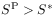the overshoot region grows linearly with time at the rate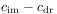. [2334.3.6] Such an overshoot solution is generated by the second initial condition B and compared with the travelling wave solution in Figure 2. [2334.3.7] The non-travelling overshoot for initial condition B with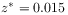and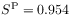is shown as the solid profiles at times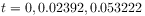corresponding to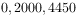s. [2334.3.8] The profile quickly approaches an overshoot solution with plateau value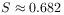close to the saturation of the Welge tangent construction. [2334.3.9] This is higher than the plateau value. [2334.3.10] The difference will be difficult to observe experimentally because of the large uncertainties in the measurements [54, 55]. [2334.3.11] The velocities of the numerical imbibition and drainage fronts in the case of initial conditions B withand(solid curves in Fig. 2) again agree perfectly with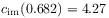and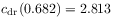from the theoretical analysis.

[2334.3.12] Equation (29) with initial and boundary conditions for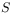and an initial condition for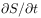is conjectured to be a well defined semigroup of bounded operators on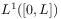on a finite interval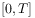of time. [2334.3.13] The conjecture is supported by the fact that each of the equations (23) individually defines such a semigroup, and because multiplication byor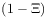are projection operators.

[2334.3.14] Equation (29) with initial and boundary conditions forand an initial condition foris conjectured to be a well defined semigroup of bounded operators onon a finite intervalof time. [2334.3.15] The conjecture is supported by the fact that each of the equations (23) individually defines such a semigroup, and because multiplication byorare projection operators.

[2334.4.1] Other values of the initial saturation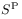have also been investigated. [2334.4.2] A rich phenomenology of possibilities indicates that the existence or nonexistence of overshoot solutions depends very sensitively on the parameters of the problem and the initial conditions.

[2334.5.1] Note also the asymmetric shape of the overshoot solutions (travelling or not). [2334.5.2] This asymmetry resembles the asymmetry seen in experiment [54, 55]. [2334.5.3] While the leading imbibition front is steep, the trailing drainage front is more smeared out. [2334.5.4] This results from the capillary flux termwhose values atare smaller than at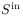.Figure 2: Numerical solutions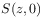,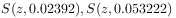of eq.  (29) at dimensionless timeswith parameters from Table 1 for initial condition A (dashed) and B (solid). For case A (shown as a short dashed line) the profile foris the same as in Fig.1c). For the solid profiles (case B) the imbibition front moves with speed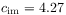while the trailing drainage front moves with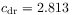.